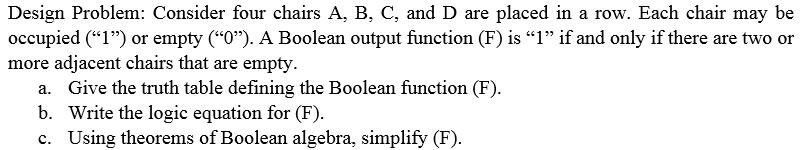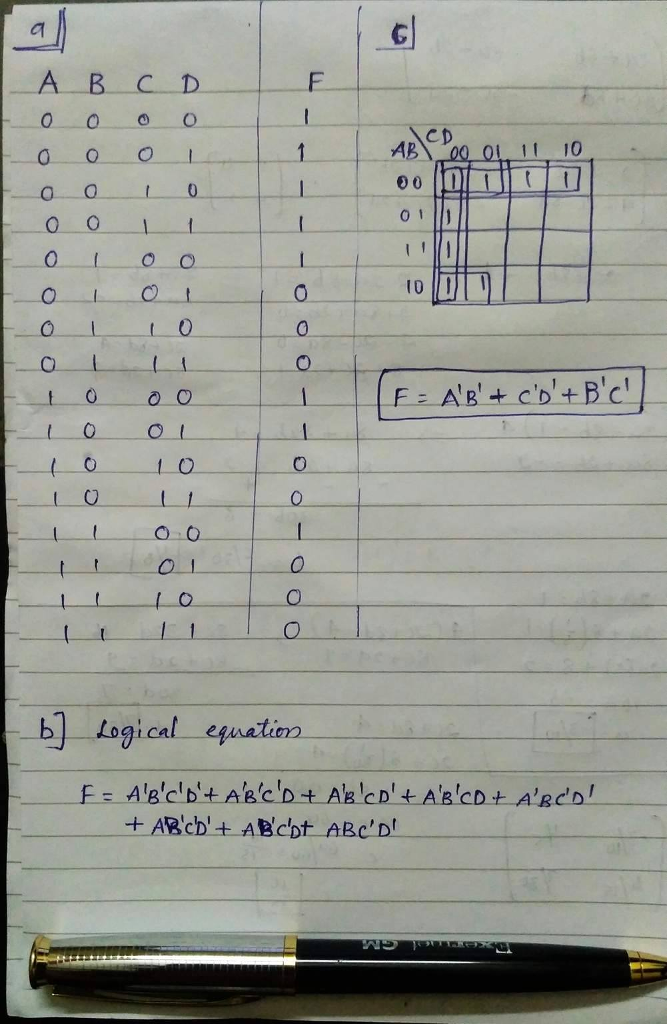# Homework Solution: Consider four chairs A, B, C, and D are placed in a row. Each chair may be occupied ("1") or empt…Consider four chairs A, B, C, and D are placed in a row. Each chair may be occupied ("1") or empty ("0"). A Boolean output function (F) is "1" if and only if there are two or more adjacent chairs that are empty. a. Give the truth table defining the Boolean function (F). b. Write the logic equation for (F). c. Using theorems of Boolean algebra, simplify (F).